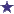# Volume/Volume Compounding - Solution Alligation Question

by janel
(us)Combining Solutions With Different Ratio Concentrations - Alligation

Prepare 480mL of a 1:30 solution using a 1:10 solution and a 1:50 solution. How many mL of each solution is needed?

### Comments for Volume/Volume Compounding - Solution Alligation Question

Average RatingRatingAllegation problem by: Shobhna This is an Alligation problem,So 1:30= 0.031:10= 0.11:50=0.02Now solve the problem:.03 - 02 =.01.1 - .03= .07Total part .08So 480ml of 1:30 is: 480*.07/.08=420 ml 6 parts480*.01/.08= 60 ml. 1 partTo make 480ml of 1:30 we have to add 7 parts of 1:50 and 1 part of 1:10 that's how we get our answer